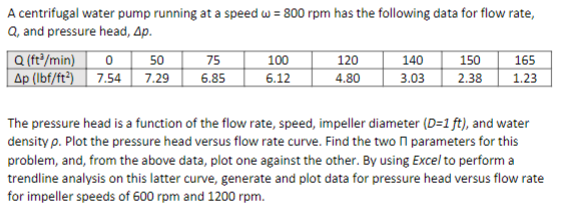# Question A centrifugal water pump running at a speed w = 800 rpm has the following data for flow rate, Q, and pressure head, Ap. Q (ft/min) O 50 75 100 120 140 150 165 Ap (lbf/ft?) 7.54 7.29 6.85 6.12 4.80 3.03 2.38 1.23 The pressure head is a function of the flow rate, speed, impeller diameter (D=1 ft), and water density p. Plot the pressure head versus flow rate curve. Find the two parameters for this problem, and, from the above data, plot one against the other. By using Excel to perform a trendline analysis on this latter curve, generate and plot data for pressure head versus flow rate for impeller speeds of 600 rpm and 1200 rpm.TH9MQL The Asker · Mechanical EngineeringTranscribed Image Text: A centrifugal water pump running at a speed w = 800 rpm has the following data for flow rate, Q, and pressure head, Ap. Q (ft/min) O 50 75 100 120 140 150 165 Ap (lbf/ft?) 7.54 7.29 6.85 6.12 4.80 3.03 2.38 1.23 The pressure head is a function of the flow rate, speed, impeller diameter (D=1 ft), and water density p. Plot the pressure head versus flow rate curve. Find the two parameters for this problem, and, from the above data, plot one against the other. By using Excel to perform a trendline analysis on this latter curve, generate and plot data for pressure head versus flow rate for impeller speeds of 600 rpm and 1200 rpm.
More
Transcribed Image Text: A centrifugal water pump running at a speed w = 800 rpm has the following data for flow rate, Q, and pressure head, Ap. Q (ft/min) O 50 75 100 120 140 150 165 Ap (lbf/ft?) 7.54 7.29 6.85 6.12 4.80 3.03 2.38 1.23 The pressure head is a function of the flow rate, speed, impeller diameter (D=1 ft), and water density p. Plot the pressure head versus flow rate curve. Find the two parameters for this problem, and, from the above data, plot one against the other. By using Excel to perform a trendline analysis on this latter curve, generate and plot data for pressure head versus flow rate for impeller speeds of 600 rpm and 1200 rpm.